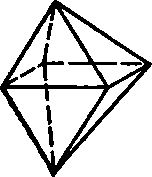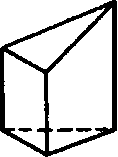A polyhedron is a solid bounded by planes. The bounding planed are called faces and their intersections are called edges. The intersections of the edges are called vertices.

A polyhedron having four faces is called a tetrahedron; one having six faces, a hexahedron; one having eight faces, and octahedron, Fig. 68; one having twelve faces, a dodecahedron, etc.

Prisms

A prism is a polyhedron having two opposite faces, called bases, which are equal and parallel, and other faces, called lateral faces, which are parallelograms, Fig. 69. The altitude of a prism is the perpendicular distance between the bases. The area of the lateral faces is called the lateral area.Fig. 68. Octahedron.Fig. 69. Prism.Fig. 70. Right Prism.

Prisms are called triangular, rectangular, hexagonal, etc., according to the shape of the bases. Further classifications are as follows:Fig. 71. Parallelopiped.Fig. 72. Rectangular Paral-lelopiped.Fig. 73. Truncated Prism.

A right prism is one whose lateral faces are perpendicular to the bases, Fig. 70.

A regular prism is a right prism having regular polygons for bases.

Parallelopiped

A parallelopiped is a prism whose bases are parallelograms, Fig. 71. If all the edges are perpendicular to the bases, it is called a right parallelopiped.

A rectangular parallelopiped is a right parallelopiped whose bases and lateral faces are rectangles, Fig. 72.

A cube is a rectangular parallelopiped all of whose faces are squares.

A truncated prism is the portion of a prism included between the base and a plane not parallel to the base, Fig. 73.

Pyramids

A pyramid is a polyhedron whose base is a polygon and whose lateral faces are triangles having a common vertex called the vertex of the pyramid.Fig. 74. Pyramid.Fig. 75. Regular Pyramid.Fig. 76. Frustum of Pyramid.

The altitude of the pyramid is the perpendicular distance from the vertex to the base.

Pyramids are named according to the kind of polygon forming the base, viz, triangular, quadriangular, Fig. 74. pentagonal, Fig. 75, hexagonal.

A regular pyramid is one whose base is a regular polygon and whose vertex lies in a perpendicular erected at the center of the base, Fig. 75.

A truncated pyramid is the portion of a pyramid included between the base and a plane not parallel to the base.

A frustum of a pyramid is the solid included between the base and a plane parallel to the base, Fig. 76; its altitude is the perpendicular distance between the bases.